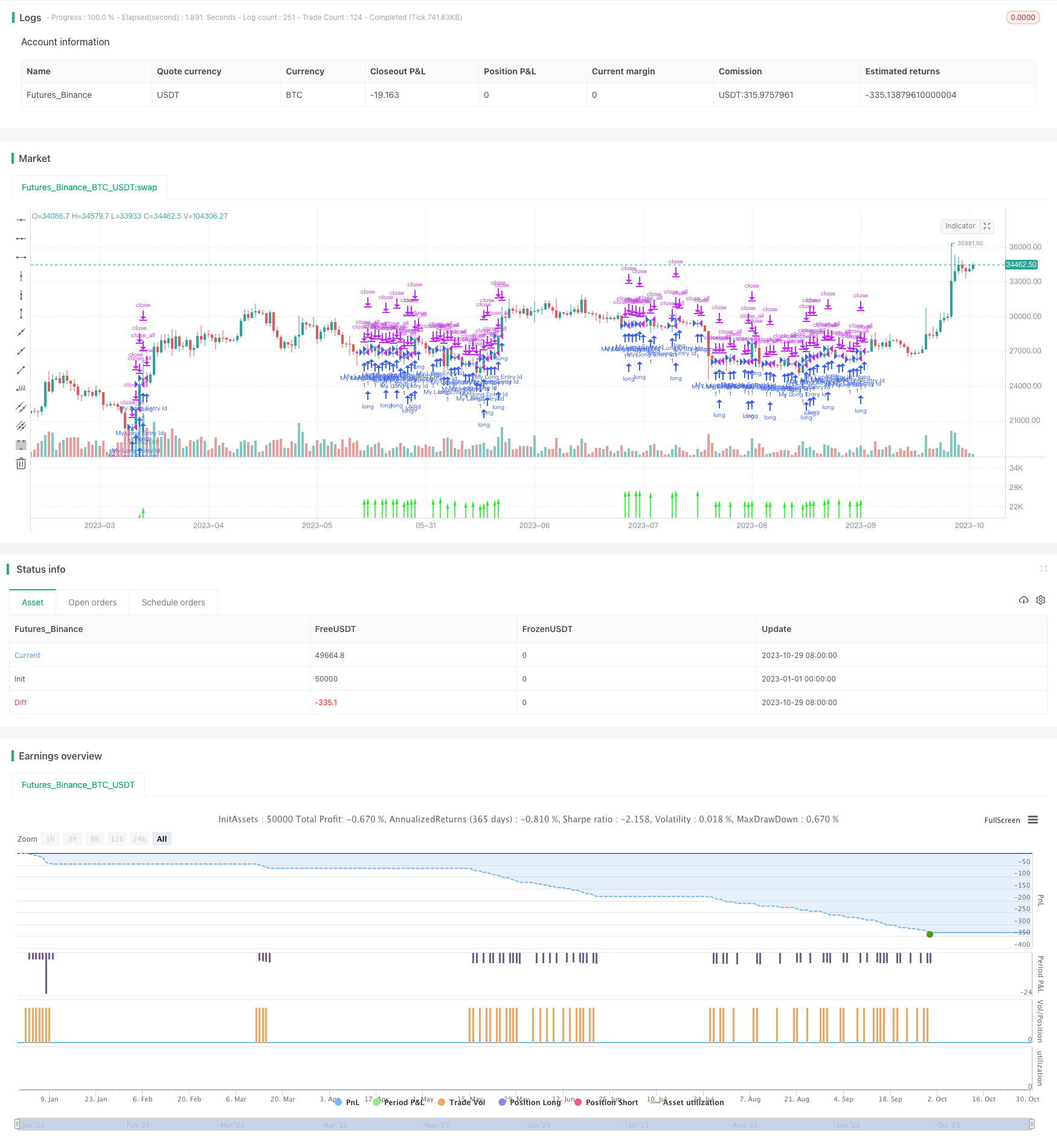# Golden Trend Tracking Strategy Based on Periodic Investment

Author: ChaoZhang, Date: 2023-10-31 15:09:22
Tags:## Overview

This strategy uses moving average models to determine market trend direction. When a bullish trend is identified, it will periodically open long positions with fixed amounts to track the uptrend of golden cross in the market.

## Strategy Logic

The strategy is mainly based on the following technical principles:

1. Use EMA lines to determine market trend direction. When the fast EMA line crosses over the slow EMA line, it is judged as a bullish trend and prepares to enter long positions.

2. Combine the MACD indicator to determine entry timing. When MACD turns from positive to negative, it indicates that buying power starts to weaken, so it’s time to enter long positions.

3. Limit to only enter once per month to avoid chasing highs. The entry amount each time can be fixed.

4. Allow setting a start date and end date to limit the backtest period. When backtest ends, the strategy will close all positions.

Specifically, the strategy first calculates the fast EMA line and slow EMA line, and detects golden cross between them to determine the market trend. At the same time, it calculates the MACD indicator to determine specific entry point. When both criteria are met, a long signal is generated. According to the rule of only entering once per month, actual entry orders are determined. The capital amount for each entry can be preset. When the backtest ends, the strategy will actively close all positions.

This is a simple and direct trend following strategy with the following advantages:

1. Using EMA lines to determine the major trend is simple and practical. EMA has a smoothing effect on price changes and can filter out market noise effectively.

2. The MACD indicator can identify the turning point when buying power starts to weaken relatively accurately, making entries safer.

3. Limiting to only chase the uptrend once per month can avoid chasing highs and killing the uptrend in a bull market.

4. Allow customizing the entry amount each month provides flexibility in position sizing.

5. Backtest can be used to evaluate strategy performance by setting a start date and end date.

6. It will close out all positions automatically when backtest ends, avoiding awkward remaining positions.

## Risks and Mitigations

There are some potential risks of this strategy:

1. Trend determination via moving averages may miss opportunities during temporary pullbacks or react slowly on trend reversal. The period can be shortened or more indicators can be added.

2. Only entering once per month may miss better entry opportunities. Consider loosening the frequency or adding another entry when breaking recent highs.

3. There are risks of curve fitting. More parameter tuning space should be allowed and robustness should be tested across markets and time periods.

4. There are risks of chasing momentum and overbuying. The monthly entry amount should be controlled to avoid oversized positions.

## Enhancement Opportunities

This periodic investment trend following strategy can be further extended and enhanced from the following aspects:

1. Add stop loss logic to actively cut losses when a bearish reversal pattern emerges.

2. Consider adding another buy when MACD histogram shows bullish divergence to get more exposure to the uptrend.

3. Introduce comparison of current month’s new high vs previous month to assess the momentum strength.

4. Add position sizing logic. The monthly entry amount can be made adaptive based on percentage rather than fixed value.

5. Evaluate the impact of different MA combinations and MACD parameters. Find the optimal parameter set.

6. Add a trailing stop loss that follows the price at a certain distance after reaching new highs, allowing profits to run.

## Summary

This strategy represents a simple and clean trend following approach using periodic investment and moving averages. It is easy to understand and implement, serving as a good starting point for learning algorithmic trading. But in live trading, position sizing needs to be controlled carefully. The strategy should be further enhanced to adapt to complex market conditions.

```/*backtest
start: 2023-01-01 00:00:00
end: 2023-10-30 00:00:00
period: 1d
basePeriod: 1h
exchanges: [{"eid":"Futures_Binance","currency":"BTC_USDT"}]
*/

// This source code is subject to the terms of the Mozilla Public License 2.0 at https://mozilla.org/MPL/2.0/

//@version=4
// strategy("Buy and Hold entry finder Strategy",pyramiding=10000, overlay=true,initial_capital=0,default_qty_type=strategy.cash,default_qty_value=1000,currency = currency.EUR,commission_type=strategy.commission.cash_per_order,commission_value=0)

//INPUTS##################################################################################################################

maxEmaDistance = input(title="Maximum EMA Distance", type=input.float, step=0.01, defval=50000)
emalength = input(title="EMA Length", type=input.integer,defval=200)

// Make input options that configure backtest date range
startDate = input(title="Start Date", type=input.integer,
defval=1, minval=1, maxval=31)
startMonth = input(title="Start Month", type=input.integer,
defval=1, minval=1, maxval=12)
startYear = input(title="Start Year", type=input.integer,
defval=2020, minval=1800, maxval=2100)

endDate = input(title="End Date", type=input.integer,
defval=12, minval=1, maxval=31)
endMonth = input(title="End Month", type=input.integer,
defval=02, minval=1, maxval=12)
endYear = input(title="End Year", type=input.integer,
defval=2021, minval=1800, maxval=2100)

endDate1=endDate-1
//starttag
//startmonat
//MACD########################################################################################################################

fast_length=12
slow_length=26
src=close
col_macd=#0094ff
fast_ma = ema(src, fast_length)
slow_ma = ema(src, slow_length)
macd = fast_ma - slow_ma

//EMA Distance CALC########################################################################################################

ma1 =ema(close,emalength)
distFromMean = close - ma1

inDateRange = true

longCondition = (distFromMean<=maxEmaDistance and distFromMean>=distFromMean and macd<=0 and inDateRange)
longnow=false

if(longCondition and strategy.position_size == 0)
strategy.entry("My Long Entry Id", strategy.long)
longnow:=true

if(longCondition and strategy.position_size > 0)
longnow:=true

if(longCondition and strategy.position_size > 0 and month>valuewhen(longnow, month ,1) or longCondition and strategy.position_size > 0 and year>valuewhen(longnow, year ,1) and inDateRange)
strategy.entry("My Long Entry Id", strategy.long)

plotchar(minute, "Minuten", "", location = location.top)

plotchar(hour, "Stunden", "", location = location.top)

plotchar(dayofmonth, "Tage", "", location = location.top)

plotchar(month, "Monat", "", location = location.top)

plotchar(year, "Jahr", "", location = location.top)

plotchar(strategy.position_size, "Positionen", "", location = location.top)

plotchar(longCondition, "Long Condition", "", location = location.top)

if true
strategy.close_all()

//#########################################################################################################################

plotArrow = if (distFromMean<=maxEmaDistance and distFromMean>=distFromMean and macd<=0)
1
else
0

plotarrow(series=plotArrow)

```

More